You are on the Home/Excel/Charts/3D Surface pageShare Your

What does the final graph look like?

Create a table of data for the chart

Create the chart

What Excel really does and why a 3-D surface chart is not a 'true' 3-D chart

 The goalPlot z=(x-10)3 + (y-100)2 as in the chart on the rightEnter the function to be graphed To ease understanding of how Excel creates a 3-D surface chart, we will start by naming the cells that will contain the x, y, and z values.  Enter the text x, y, and z in cells A1:A3 as on the right.  Then, select A1:B3 and select the menu item Insert | Name > Create...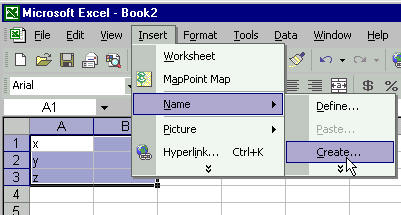Accept Excel's default choice of 'Left column' by clicking the OK button.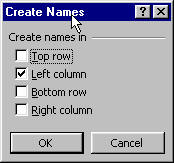Now, enter the function in cell B3 as shown on the right.  Note that the formula uses the names of the cells, rather than the cell addresses.  If we had not named the cells, the formula might have looked like=(B1-10)^3+(B2-100)^2Create the data table for the chart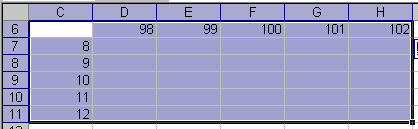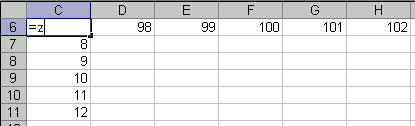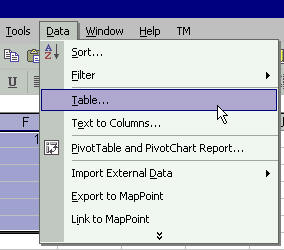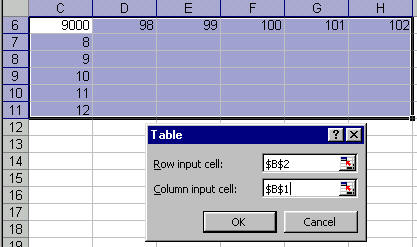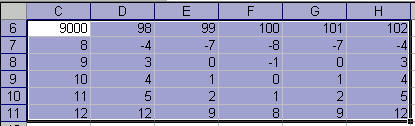Create the chart Step 1: The basic chart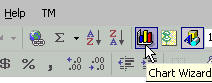Step 2: Specify the 'x-values'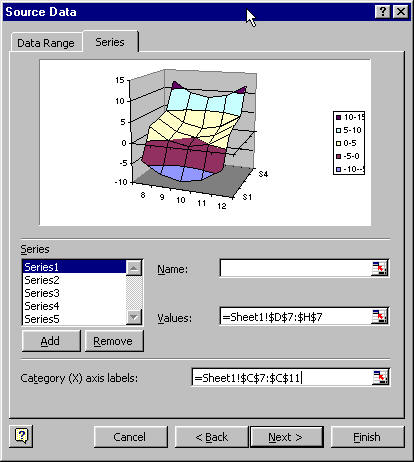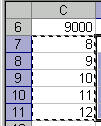Step 3: Specify the 'y-values'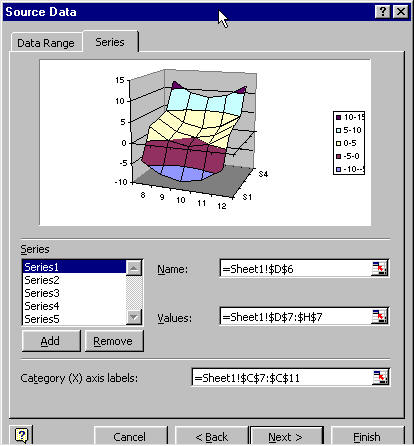Step 4: The result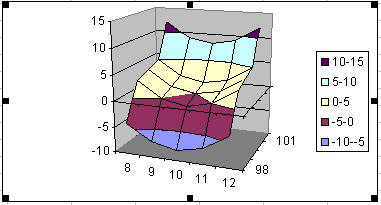What's the limitation on creating a 3-D chart? In creating the chart above, the x-values were entered not a real numeric values but as 'category labels.'  This was done in Step 2 of the Chart Wizard.  Excel does not attach any numerical significance to these labels.  All the labels are arranged so that they are next to each other at equal distances. Similarly, the y-values are not real values.  Excel draws a 3-D chart by charting multiple series and overlaying a surface across all the series.  What looks like y-values of 98, 99, etc., are nothing but series names specified in Step 2 of the Chart Wizard. This example deliberately picked x-values such that the difference between adjacent values was the same (1 in this case).  The y-values have the same characteristic, with each being 1 more than the previous one. Any category chart which contains equidistant x-values will look OK.  However, consider the case where the x values are 8, 9, 10, 15, and 20.  The chart corresponding to this data set is shown on the right.